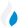•Trade Pi
•Trade
•Exchange
•Trade Pi
•Trade
•Exchange
\$1.09T
Total marketcap
\$79.1B
Total volume
41.04%
BTC dominance
•Trade Pi
•Trade
•Exchange

# PERPI to WETH Exchange Rate - 1 Perp Inu in WETH

0.00
Vol [24h]
\$1,129.48

## perpi to weth converter

Exchange Pair Price 24h volume
Uniswap (Arbitrum One) PERPI/WETH \$0.1347 \$1.13K

## PERPI/WETH Exchange Rate Overview

Name Ticker Price % 24h 24h high 24h low 24h volume
Perp Inu perpi \$0.1346 -4.0859% \$0.1436 \$0.1345 \$1.13K
WETH weth \$1,583.59 -2.1943% \$1,656.78 \$1,572.27 \$1.19B

Selling 1 PERPI you get 0.00 WETH weth.

Perp Inu (not set) had the highest price, at that time trading at its all-time high of \$0.

0 days have passed since then, and now the price is 0 of the maximum.

Based on the table data, the PERPI vs WETH exchange volume is \$1,129.48.

Using the calculator/converter on this page, you can make the necessary calculations with a pair of Perp Inu/WETH.

## Q&A

### What is the current PERPI to WETH exchange rate?

Right now, the PERPI/WETH exchange rate is 0.00.

### What has been the Perp Inu to WETH trading volume in the last 24 hours?

Relying on the table data, the Perp Inu to WETH exchange volume is \$1,129.48.

### How can I calculate the amount of WETH? / How do I convert my Perp Inu to WETH?

You can calculate/convert WETH from Perp Inu to WETH converter. Also, you can select other currencies from the drop-down list.

## PERPI to WETH Сonversion Table

PERPI WETH
4 PERPI = 0.0003 WETH
7 PERPI = 0.0005 WETH
20 PERPI = 0.001 WETH
30 PERPI = 0.002 WETH
40 PERPI = 0.003 WETH
80 PERPI = 0.006 WETH
200 PERPI = 0.01 WETH
400 PERPI = 0.03 WETH
800 PERPI = 0.06 WETH
8000 PERPI = 0.6 WETH
80000 PERPI = 6.8 WETH
800000 PERPI = 68.06 WETH
8000000 PERPI = 680.6 WETH